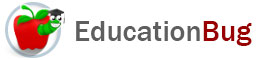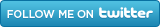PUBLIC SCHOOLS PRIVATE SCHOOLS SCHOOL DISTRICTS COLLEGES PUBLIC LIBRARIES JOBS BLOG RESOURCES
Career Education
Education Choices
Education Costs
Education History and General Information
Education Issues
Education Resources
Family Involvement in Education
Students w Special Needs
Subject Information and Homework Help
Teaching and Learning
Testing
By Types of Schools
By Specific Audiences
GeneralPerimeter

In this article about perimeter, what perimeter is and how to calculate perimeter are both explained. Learn how to find the perimeter of a polygon, whether equilateral or not, and how to find both the perimeter, or circumference, and area of a circle.

The word perimeter originated in Greek and came into English through Latin and Middle English. The meaning in Greek was â€œmeasure around,â€ and thatâ€™s very close to both its specialized meaning in math and its more general, common meaning. For more about perimeter, continue reading this article.

Perimeter in Generic Terms

When weâ€™re not talking about the field of mathematics, the perimeter refers to the boundary around something two-dimensional or three-dimensional. We might speak of the perimeter of a building, or a path that goes around the perimeter of a park, as opposed to cutting across the middle of the park. In this context, perimeter is a rough outline of a shape or solid mass.

Perimeter in the Field of Mathematics

In the world of math, the concept of perimeter is more specifically defined. The perimeter is the closed boundary of a plane area, that is, the edge of a geometric shape (for more about them, see the article â€œGeometric Shapes.â€) or a plane shape: a closed shape in two dimensions. Finding the perimeter of a figure means determining the distance around this boundary. It is found by measuring, adding known measurements, or computing the distance or segments of the distance based on other known information.

Perimeter of Polygons

Polygons are plane figures with a closed perimeter that includes a finite number of line segments. A polygon must have a minimum of three sides to meet the criteria in the preceding sentence, so the polygon with the minimum number of sides would be a triangle. Quadrilaterals, pentagons, hexagons, heptagons, and octagons, are polygons with four, five, six, seven, and eight sides respectively.

Regular polygons, also called equilateral polygons, have sides of equal length, so if you know the length of one side, you can compute the perimeter by multiplying that length by the number of sides that the polygon has. This can be written:

p = a(n)

where p is the perimeter, a is the length of one side and n is the number of sides. Equilateral polygons include equilateral triangles and the quadrilaterals known as rhombuses, of which the square is an example.

If the polygon does not have sides of equal length, then you can add the length of the sides, using the model:

p = a + b + c . . .

where p is the perimeter and a, b, c, etc. are the lengths of the sides.

Circles

A circle is a plane figure for which all the points on the perimeter are a fixed distance from the center point. This fixed distance is called the radius, and the distance from any point on the perimeter, connected by a straight line segment drawn through the center of the circle to another point on the perimeter on the opposite side is called the diameter. The perimeter of a circle is called the circumference. It is the only polygon to have a different name for its perimeter.

The perimeter of a circle is found by a different type of formula than the method of finding the perimeter for polygons. It is written:

c = 2p r

where c is the circumference, p is the constant pi, having a value of 3.14159. . . and r is the length of the radius. Because of the nature of a circle, the radius and the circumference always have a fixed relationship, and the constant p is an expression of that relationship.

Because the diameter is twice the length of the radius, it can also be written:

c = p d

where d is the diameter. Some people may prefer to use this second expression of the circumference of a circle to help them prevent confusion with the formula for the area of a circle, which is so similar to the circumference formula that many people confuse them.

The area formula is this:

A = Ï€r2

where A is the area, Ï€ is pi, and r2 is the radius squared (multiplied by itself).